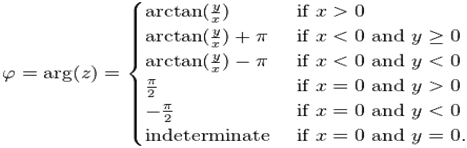Click to Chat

1800-1023-196

+91-120-4616500

CART 0

• 0

MY CART (5)

Use Coupon: CART20 and get 20% off on all online Study Material

ITEM
DETAILS
MRP
DISCOUNT
FINAL PRICE
Total Price: Rs.

There are no items in this cart.
Continue Shopping• Complete JEE Main/Advanced Course and Test Series
• OFFERED PRICE: Rs. 15,900
• View Details

```Revision Notes on Complex Numbers

z1 = a+ib and z2 = c+id then z1 = z2 implies that a = c and b = d.

If we have a complex number z where z = a+ib, the conjugate of the complex number is denoted by z* and is equal to a-ib. In fact, for any complex number z, its conjugate is given by z* = Re(z) – Im(z).

Division of complex numbers: The numerator as well as denominator should first be multiplied by the conjugate of the denominator and then simplified.

Argument of a complex number:

1. Argument of a complex number p(z) is defined by the angle which OP makes with the positive direction of x-axis.

2. Argument of z generally refers to the principal argument of z (i.e. the argument lying in (–π, π) unless the context requires otherwise.

3. Hence, the argument of the complex number z = a + ib = r (cos θ + i sin θ) is the value of θ satisfying r cos θ = a and r sin θ = b.

4. The angle θ is given by θ = tan-1 |b/a|.

5. The value of argument in various quadrants is given below:If OP = |z| and arg (z) = θ, then obviously z = r (cos θ + i sin θ) and is called the polar form of complex number z.

|(z-z1) / (z-z2)| =1 the locus of point representing z is the perpendicular bisector of line joining z1 and z2.

-|z| ≤ Re(z) ≤ |z| and -|z| ≤ Im(z) ≤ |z|

If a and b are real numbers and z1 and z2 are complex numbers then

|az1 + bz2|2 + |bz1 - az2|2 = (a2 + b2) (|z1|2 + (|z2|2)

The distance between the complex numbers z1 and z2 is given by |z1 - z2|.

In parametric form, the equation of line joining z1 and z2 is given by z = tz1 + (1-t)z2.

If A(z1) and B(z2) are two points in the argand plane, then the complex slope μ of the straight line AB is given by μ = (z1 - z2)/ (1 - 2).

Two lines having complex slopes μ1 and μ2 are:

1. Parallel iff μ1 = μ2

2. Perpendicular iff μ1 = - μ2 or μ1 + μ2 = 0

If A(z1), B(z2), C(z3) and D(z4) are four points in the argand plane, then the angle θ between the lines AB and CD is given by θ = arg{(z1 - z2)/ (z3 – z4)}
Some basic properties of complex numbers:

I. ||z1| - |z2|| = |z1+z2| and |z1-z2| = |z1| + |z2| iff origin, z1, and z2 are    collinear and origin lies between z1 and z2.

II. |z1 + z2| = |z1| + |z2| and ||z1| - |z2|| = |z1-z2| iff origin, z1 and z2 are collinear and z1 and z2 lie on the same side of origin.

III. The product of nth roots of any complex number z is z(-1)n-1.

IV. amp(zn) = n amp z

V. The least value of |z - a| + |z - b|  is |a - b|.Demoivre's Theorem: The theorem can be stated in two forms:

Case I: If n is any integer, then

(i) (cos θ + i sin θ)n = cos nθ + i sin nθ

(ii) (cos θ1 + i sin θ1) . (cos θ2 + i sin θ2) ......... (cos θn + i sin θn)

= cos (θ1 + θ2 + θ3 .................. + θn) + i sin (θ1 + θ2 + ..............  θn)

Case II: For p and q such that q ≠ 0, we have

(cos θ + i sin θ)p/q = cos((2kπ + pq)/q) + isin((2kπ+pq/q)  where k = 0,1,2,3,.....,q-1

Demoivre’s formula does not hold for non-integer powers.

Main application of Demoivre’s formula is in finding the nth roots of unity. So, if we write the complex number z in the polar form then,

z = r(cos x + isin x)

Then z1/n = [r (cos x +i sinx )]1/n

= r 1/n [ cos (x+2kπ/n) + i sin (x+2kπ/n)]

Here k is an integer. To get the n different roots of z one only needs to consider values of k from 0 to n – 1.

Continued product of the roots of a complex quantity should be determined using theory of equations.

The modulus of a complex number is given by |z| = √x2+y2.

The only complex number with modulus zero is the number (0, 0).

The following figures illustrate geometrically the meaning of addition and subtraction of complex numbers:```### Course Features

• 728 Video Lectures
• Revision Notes
• Previous Year Papers
• Mind Map
• Study Planner
• NCERT Solutions
• Discussion Forum
• Test paper with Video Solution